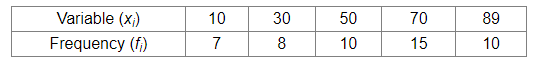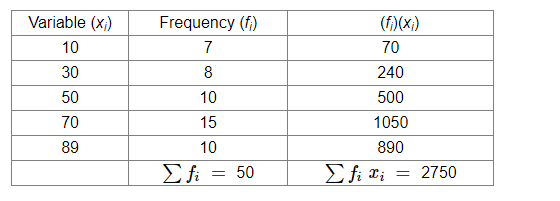# Find the mean of the following frequency distribution:Question:

Find the mean of the following frequency distribution:Solution:

We will make the following table:Thus, we have:

Mean $=\frac{\sum f_{i} x_{i}}{\sum x_{i}}$

$=\frac{2750}{50}=55$